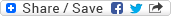## Sunday, June 1, 2008

### Potential problem involving overflow/truncation in C

Question: What's the potential problem with the followinf C code:-

int var1 = 1000;
int var 2 = 2000;
long int value = var1 * var2;

Answer: In this case, var1 and var2 both are variable of type 'int' and hence the multiplication is carried out as the multiplication of two 'int's and hence the result of the multiplication may overflow (on certain machines where int if only 2 bytes long) and in turn truncated before being assigned to the variable 'value' of type 'long'. Ironically in this case, first the truncation will happen and then the truncated value will be promoted to a 'long' value before being assigned to the variable 'value'.

How can we fix this problem?

Very easy to fix it... we just need to convert the 'int' multiplication into a 'long' multiplication and for that we just need to cast at least one (you won't like to cast two if the same can be achieved by casting only one) of the operands to type 'long'. This casting will promote both the 'int's to 'long' before the multiplication is carried out. Now, the result will be a long value and the exact value can be assigned to the variable 'value', which is of type 'long' without any truncation. So, we need to do just the following to fix the potential problem:-

...
long int value = (long)var1 * var2;
...

Okay... what'll happen if we have the right side of the assignment operator as '(long)(var1 * var2)' instead of '(long)var1 * var2'? Do we have the same results everytime? Well... the answer is NO for the abvious reason... leaving it for you :-)